# 1.5 Exponential and logarithmic functions  (Page 8/17)

 Page 8 / 17

$f\left(x\right)={e}^{x}+2$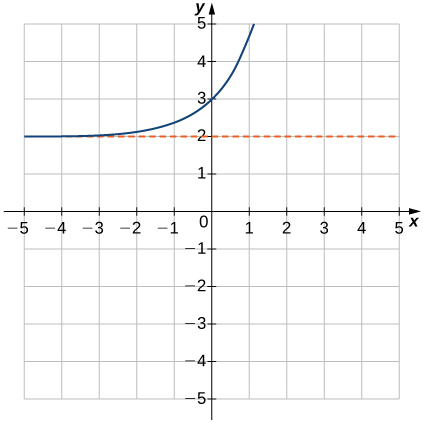Domain: all real numbers, range: $\left(2,\infty \right),y=2$

$f\left(x\right)=\text{−}{2}^{x}$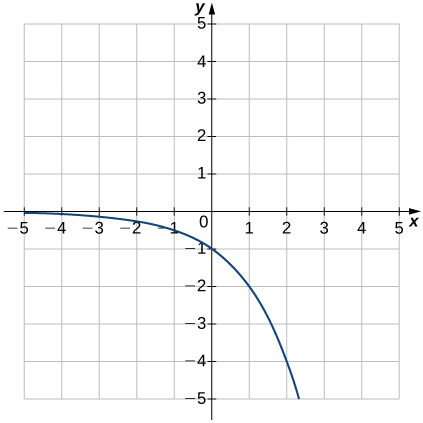$f\left(x\right)={3}^{x+1}$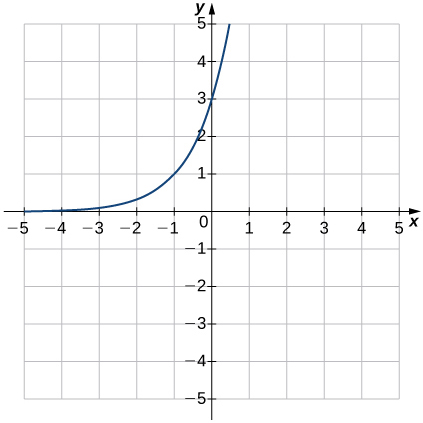Domain: all real numbers, range: $\left(0,\infty \right),y=0$

$f\left(x\right)={4}^{x}-1$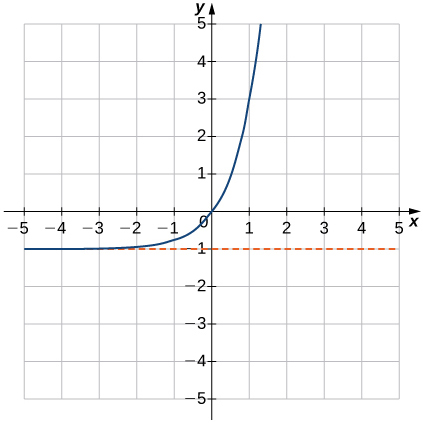$f\left(x\right)=1-{2}^{\text{−}x}$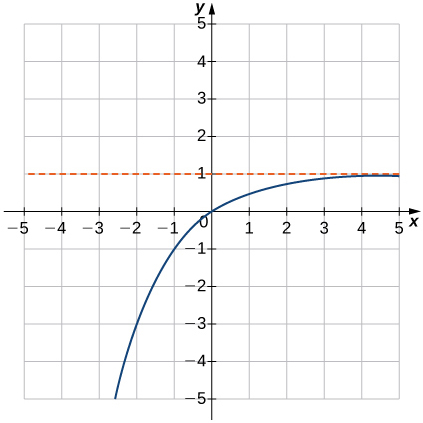Domain: all real numbers, range: $\left(\text{−}\infty ,1\right),y=1$

$f\left(x\right)={5}^{x+1}+2$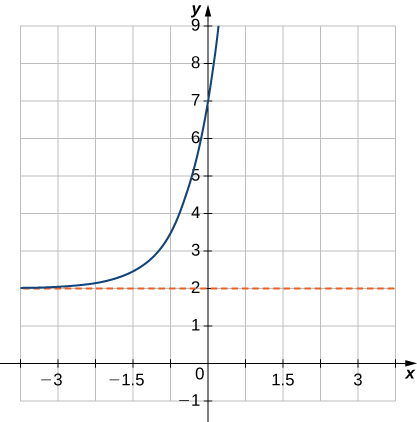$f\left(x\right)={e}^{\text{−}x}-1$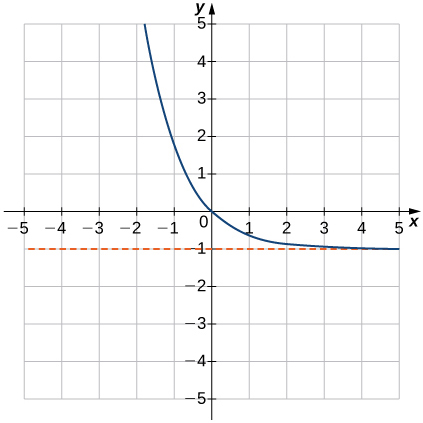Domain: all real numbers, range: $\left(-1,\infty \right),y=-1$

For the following exercises, write the equation in equivalent exponential form.

${\text{log}}_{3}81=4$

${\text{log}}_{8}2=\frac{1}{3}$

${8}^{1\text{/}3}=2$

${\text{log}}_{5}1=0$

${\text{log}}_{5}25=2$

${5}^{2}=25$

$\text{log}\phantom{\rule{0.1em}{0ex}}0.1=-1$

$\text{ln}\left(\frac{1}{{e}^{3}}\right)=-3$

${e}^{-3}=\frac{1}{{e}^{3}}$

${\text{log}}_{9}3=0.5$

$\text{ln}\phantom{\rule{0.1em}{0ex}}1=0$

${e}^{0}=1$

For the following exercises, write the equation in equivalent logarithmic form.

${2}^{3}=8$

${4}^{-2}=\frac{1}{16}$

${\text{log}}_{4}\left(\frac{1}{16}\right)=-2$

${10}^{2}=100$

${9}^{0}=1$

${\text{log}}_{9}1=0$

${\left(\frac{1}{3}\right)}^{3}=\frac{1}{27}$

$\sqrt{64}=4$

${\text{log}}_{64}4=\frac{1}{3}$

${e}^{x}=y$

${9}^{y}=150$

${\text{log}}_{9}150=y$

${b}^{3}=45$

${4}^{-3\text{/}2}=0.125$

${\text{log}}_{4}0.125=-\frac{3}{2}$

For the following exercises, sketch the graph of the logarithmic function. Determine the domain, range, and vertical asymptote.

$f\left(x\right)=3+\text{ln}\phantom{\rule{0.1em}{0ex}}x$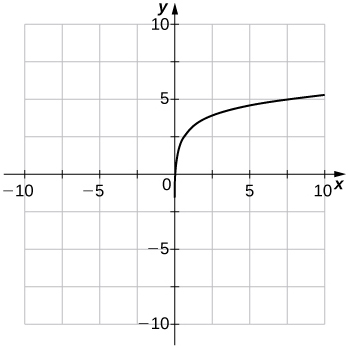$f\left(x\right)=\text{ln}\left(x-1\right)$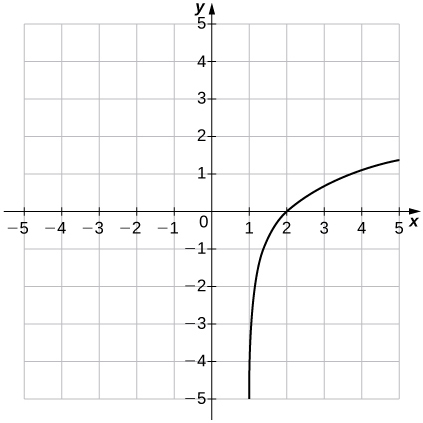Domain: $\left(1,\infty \right),$ range: $\left(\text{−}\infty ,\infty \right),x=1$

$f\left(x\right)=\text{ln}\left(\text{−}x\right)$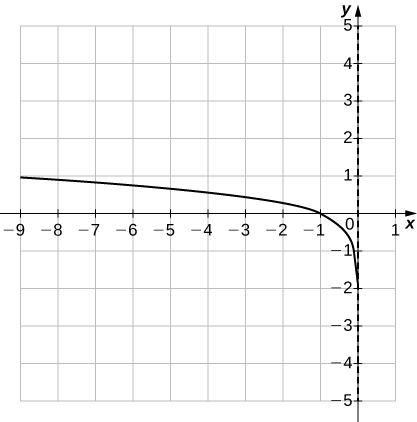$f\left(x\right)=1-\text{ln}\phantom{\rule{0.1em}{0ex}}x$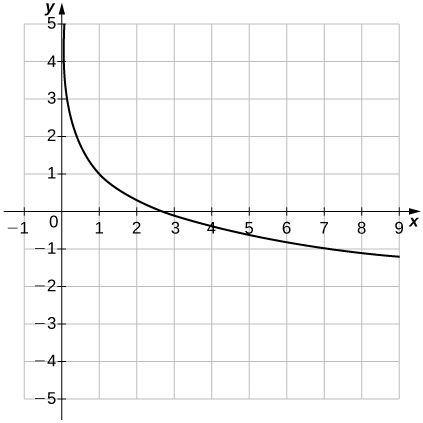Domain: $\left(0,\infty \right),$ range: $\left(\text{−}\infty ,\infty \right),x=0$

$f\left(x\right)=\text{log}\phantom{\rule{0.1em}{0ex}}x-1$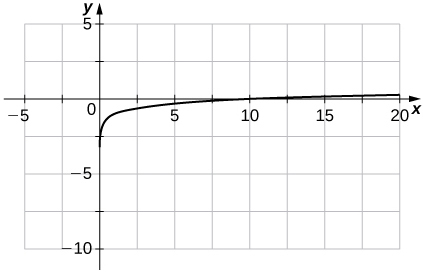$f\left(x\right)=\text{ln}\left(x+1\right)$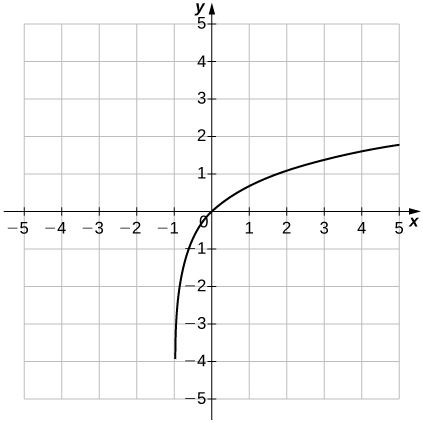Domain: $\left(-1,\infty \right),$ range: $\left(\text{−}\infty ,\infty \right),x=-1$

For the following exercises, use properties of logarithms to write the expressions as a sum, difference, and/or product of logarithms.

$\text{log}{x}^{4}y$

${\text{log}}_{3}\frac{9{a}^{3}}{b}$

$2+3{\text{log}}_{3}a-{\text{log}}_{3}b$

$\text{ln}\phantom{\rule{0.1em}{0ex}}a\sqrt{b}$

${\text{log}}_{5}\sqrt{125x{y}^{3}}$

$\frac{3}{2}+\frac{1}{2}{\text{log}}_{5}x+\frac{3}{2}{\text{log}}_{5}y$

${\text{log}}_{4}\frac{\sqrt{xy}}{64}$

$\text{ln}\left(\frac{6}{\sqrt{{e}^{3}}}\right)$

$-\frac{3}{2}+\text{ln}\phantom{\rule{0.1em}{0ex}}6$

For the following exercises, solve the exponential equation exactly.

${5}^{x}=125$

${e}^{3x}-15=0$

$\frac{\text{ln}\phantom{\rule{0.1em}{0ex}}15}{3}$

${8}^{x}=4$

${4}^{x+1}-32=0$

$\frac{3}{2}$

${3}^{x\text{/}14}=\frac{1}{10}$

${10}^{x}=7.21$

$\text{log}\phantom{\rule{0.1em}{0ex}}7.21$

$4·{2}^{3x}-20=0$

${7}^{3x-2}=11$

$\frac{2}{3}+\frac{\text{log}\phantom{\rule{0.1em}{0ex}}11}{3\phantom{\rule{0.1em}{0ex}}\text{log}\phantom{\rule{0.1em}{0ex}}7}$

For the following exercises, solve the logarithmic equation exactly, if possible.

${\text{log}}_{3}x=0$

${\text{log}}_{5}x=-2$

$x=\frac{1}{25}$

${\text{log}}_{4}\left(x+5\right)=0$

$\text{log}\left(2x-7\right)=0$

$x=4$

$\text{ln}\sqrt{x+3}=2$

${\text{log}}_{6}\left(x+9\right)+{\text{log}}_{6}x=2$

$x=3$

${\text{log}}_{4}\left(x+2\right)-{\text{log}}_{4}\left(x-1\right)=0$

$\text{ln}\phantom{\rule{0.1em}{0ex}}x+\text{ln}\left(x-2\right)=\text{ln}\phantom{\rule{0.1em}{0ex}}4$

$1+\sqrt{5}$

For the following exercises, use the change-of-base formula and either base 10 or base e to evaluate the given expressions. Answer in exact form and in approximate form, rounding to four decimal places.

${\text{log}}_{5}47$

${\text{log}}_{7}82$

$\left(\frac{\text{log}\phantom{\rule{0.1em}{0ex}}82}{\text{log}\phantom{\rule{0.1em}{0ex}}7}\approx 2.2646\right)$

${\text{log}}_{6}103$

${\text{log}}_{0.5}211$

$\left(\frac{\text{log}\phantom{\rule{0.1em}{0ex}}211}{\text{log}\phantom{\rule{0.1em}{0ex}}0.5}\approx -7.7211\right)$

${\text{log}}_{2}\pi$

${\text{log}}_{0.2}0.452$

$\left(\frac{\text{log}\phantom{\rule{0.1em}{0ex}}0.452}{\text{log}\phantom{\rule{0.1em}{0ex}}0.2}\approx 0.4934\right)$

Rewrite the following expressions in terms of exponentials and simplify.

a. $2\phantom{\rule{0.1em}{0ex}}\text{cosh}\left(\text{ln}\phantom{\rule{0.1em}{0ex}}x\right)$ b. $\text{cosh}\phantom{\rule{0.1em}{0ex}}4x+\text{sinh}\phantom{\rule{0.1em}{0ex}}4x$ c. $\text{cosh}\phantom{\rule{0.1em}{0ex}}2x-\text{sinh}\phantom{\rule{0.1em}{0ex}}2x$ d. $\text{ln}\left(\text{cosh}\phantom{\rule{0.1em}{0ex}}x+\text{sinh}\phantom{\rule{0.1em}{0ex}}x\right)+\text{ln}\left(\text{cosh}\phantom{\rule{0.1em}{0ex}}x-\text{sinh}\phantom{\rule{0.1em}{0ex}}x\right)$

[T] The number of bacteria N in a culture after t days can be modeled by the function $N\left(t\right)=1300·{\left(2\right)}^{t\text{/}4}.$ Find the number of bacteria present after 15 days.

$~17,491$

[T] The demand D (in millions of barrels) for oil in an oil-rich country is given by the function $D\left(p\right)=150·{\left(2.7\right)}^{-0.25p},$ where p is the price (in dollars) of a barrel of oil. Find the amount of oil demanded (to the nearest million barrels) when the price is between $15 and$20.

[T] The amount A of a $100,000 investment paying continuously and compounded for t years is given by $A\left(t\right)=100,000·{e}^{0.055t}.$ Find the amount A accumulated in 5 years. Approximately$131,653 is accumulated in 5 years.

[T] An investment is compounded monthly, quarterly, or yearly and is given by the function $A=P{\left(1+\frac{j}{n}\right)}^{nt},$ where $A$ is the value of the investment at time $t,P$ is the initial principle that was invested, $j$ is the annual interest rate, and $n$ is the number of time the interest is compounded per year. Given a yearly interest rate of 3.5% and an initial principle of \$100,000, find the amount $A$ accumulated in 5 years for interest that is compounded a. daily, b., monthly, c. quarterly, and d. yearly.

f(x) = x-2 g(x) = 3x + 5 fog(x)? f(x)/g(x)
fog(x)= f(g(x)) = x-2 = 3x+5-2 = 3x+3 f(x)/g(x)= x-2/3x+5
diron
pweding paturo nsa calculus?
jimmy
how to use fundamental theorem to solve exponential
find the bounded area of the parabola y^2=4x and y=16x
what is absolute value means?
Chicken nuggets
Hugh
🐔
MM
🐔🦃 nuggets
MM
(mathematics) For a complex number a+bi, the principal square root of the sum of the squares of its real and imaginary parts, √a2+b2 . Denoted by | |. The absolute value |x| of a real number x is √x2 , which is equal to x if x is non-negative, and −x if x is negative.
Ismael
find integration of loge x
find the volume of a solid about the y-axis, x=0, x=1, y=0, y=7+x^3
how does this work
Can calculus give the answers as same as other methods give in basic classes while solving the numericals?
log tan (x/4+x/2)
Rohan
Rohan
y=(x^2 + 3x).(eipix)
Claudia
Ismael
A Function F(X)=Sinx+cosx is odd or even?
neither
David
Neither
Lovuyiso
f(x)=1/1+x^2 |=[-3,1]
apa itu?
fauzi
determine the area of the region enclosed by x²+y=1,2x-y+4=0
Hi
MP
Hi too
Vic
hello please anyone with calculus PDF should share
Which kind of pdf do you want bro?
Aftab
hi
Abdul
can I get calculus in pdf
Abdul
explain for me
Usman
How to use it to slove fraction
Hello please can someone tell me the meaning of this group all about, yes I know is calculus group but yet nothing is showing up
Shodipo
You have downloaded the aplication Calculus Volume 1, tackling about lessons for (mostly) college freshmen, Calculus 1: Differential, and this group I think aims to let concerns and questions from students who want to clarify something about the subject. Well, this is what I guess so.
Jean
Im not in college but this will still help
nothing
how en where can u apply it
Migos
how can we scatch a parabola graph
Ok
Endalkachew
how can I solve differentiation?
with the help of different formulas and Rules. we use formulas according to given condition or according to questions
CALCULUS
For example any questions...
CALCULUS
v=(x,y) وu=(x,y ) ∂u/∂x* ∂x/∂u +∂v/∂x*∂x/∂v=1
log tan (x/4+x/2)
Rohan
what is the procedures in solving number 1?By OpenStaxBy OpenStaxBy Rohini AjayBy OpenStaxBy Michael SagBy Jazzycazz JacksonBy Jonathan LongBy OpenStaxBy OpenStaxBy OpenStax